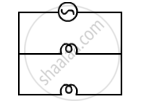Share

# Two Lamps, One Rated 40 W at 220 V and the Other 60 W at 220 V, Are Connected in Parallel to the Electric Supply at 220 V. (A) Draw a Circuit Diagram to Show the Connections. (B - CBSE Class 10 - Science

#### Question

Two lamps, one rated 40 W at 220 V and the other 60 W at 220 V, are connected in parallel to the electric supply at 220 V.

(a) Draw a circuit diagram to show the connections.
(b) Calculate the current drawn from the electric supply.
(c) Calculate the total energy consumed by the two lamps together when they operate for one hour.

#### Solution

(a)    220 V(b) Current drawn by the 40 W bulb, I_1=P/V=40/200=0.18A

Current drawn by the 60 W bulb, I_2=P/V=60/200=0.27A

Total current drawn, I=I_1+I_2=0.18A+0.27A=0.45A

(c) Total energy consumed, E=sum(pxxt)=(40xx1)+(60xx1)=100wh=0.1"kwh""

Is there an error in this question or solution?

#### Video TutorialsVIEW ALL 

Solution Two Lamps, One Rated 40 W at 220 V and the Other 60 W at 220 V, Are Connected in Parallel to the Electric Supply at 220 V. (A) Draw a Circuit Diagram to Show the Connections. (B Concept: Electric Current and Circuit.
S